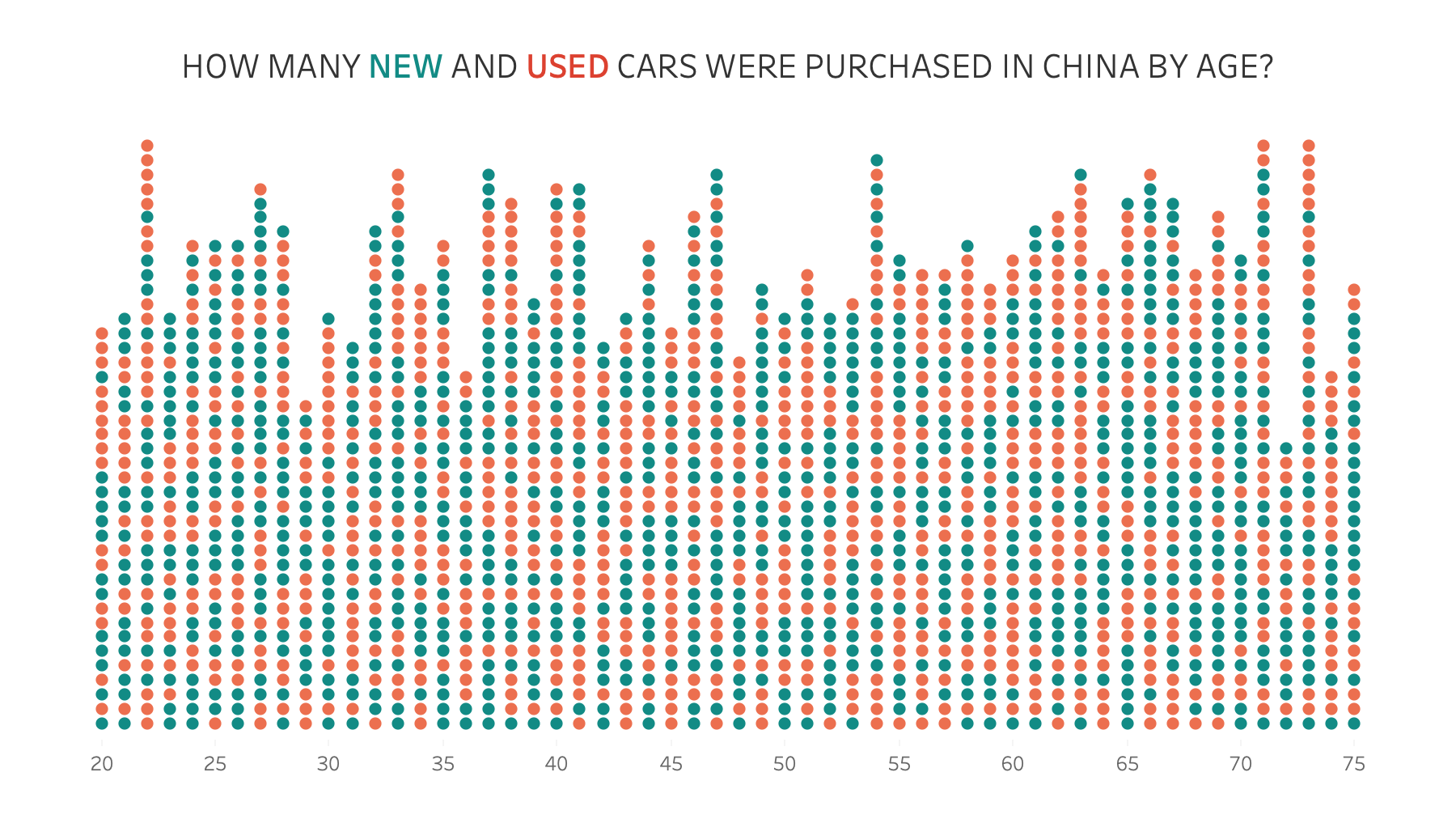Data Viz Done Right

# How to Create a Dot Matrix PlotDot matrix charts are useful for visualizing the distribution and frequency of discrete data. They help you understand the scale and proportion. The purchase is to understand how many, not how much.

In this example, I'm using two colors to distinguish new vs. used cars. However, if you have only one variable or category, then stick to one color.

Resources:

1. Workbook - https://bit.ly/DotMatrixPlot
2. Data set - https://data.world/vizwiz/car-sales-mock-data